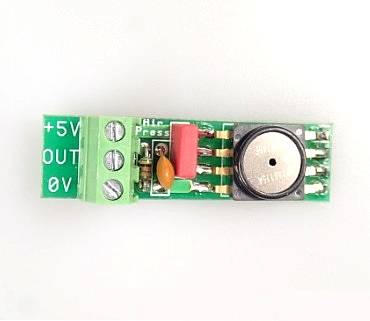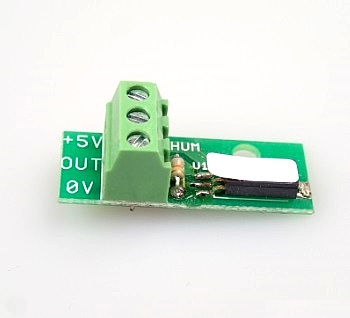24 - 01 - 2021# Block AltonaSensorTemp

AltonaLab Temperature sensor works in range -20..+100 C, accuracy: 1%. The board has a connector with three screws – ground, power +5V and signal output.The measured output voltage Vout of the sensor can be converted to temperature using the expression below:

T = (Vout-2.7315)*100.0

In case, the Vout is measured with ADC 0..3.3V, it would be good to be used a connector board with a resistor divider 1.5k/3.0k.

The used sensor element is LM335.

Instead of an expression, in AltonaLab diagram can be used a functional block AltonaSensorTemp:

Parameters:

• Input type - this parameter determines the type of an input of the block In. The parameter opens an interface with a few elements:

- Combo box Input type has two values - Raw data and Volts. In case Raw data is set, the input In is in ADC range, which depends on the next combo box ADC resolution: for 10bit ADC the input range is 0..1023. In case Volts is set, then the input In of the block is in voltage.

- Combo box ADC max range - can be set as 3.3V or 5.0V. For ADC with 3.3V max range, a resistor divider 1.5k/3.0k has to be used to change the sensor output level from 0..5V to ADC input level 0..3.3V. At this case, the functional block will calculate the right temperature value;

• Offset - the parameter moves the output value of the block with value of the parameter. Can be used for adjustment.
• QueueSize - determines how much old measured values of the temperature to be saved at the block's memory. The output of the block will be an average value of the last measured values.

Block's inputs:

• In - input value of the block, can be in ADC raw data 0..1023 or in volts;
• Process - if the input is connected, the block will calculate the input value In to temperature only at low to high front of this input. If the input is not connected, the block will calculate the input signal always.

Block's outputs:

• Temp - the calculated temperature;
• OnReady - when the calculation of the block is ready, this output becomes to a short in time high level;# Block AltonaSensorAirPress

AltonaLab Air pressure sensor works in range -15kPa ~ 115kPa, accuracy: 1.5%. The board has a connector with three screws – ground, power +5V and signal output. The measured output voltage Vout of the sensor can be converted to kPa pressure using the expression below:

P = Vout/0.0045 + 100.55

In case, the Vout is measured with ADC 0..3.3V, additional connector board is needed with a resistor divider 1.5k/3.0k.

The used sensitive element is MPXA6115A6U. It has an operating temperature range -40C..+125C and is internally temperature compensated:Instead of an expression, in AltonaLab diagram can be used a functional block AltonaSensorAirPress:

Parameters:

• Input type - this parameter determines the type of an input of the block In. The parameter opens an interface with a few elements:

- Combo box Input type has two values - Raw data and Volts. In case Raw data is set, the input In is in ADC range, which depends on the next combo box ADC resolution: for 10bit ADC the input range is 0..1023. In case Volts is set, then the input In of the block is in voltage.

- Combo box ADC max range - can be set as 3.3V or 5.0V. For ADC with 3.3V max range, a resistor divider 1.5k/3.0k has to be used to change the sensor output level from 0..5V to ADC input level 0..3.3V. At this case, the functional block will calculate the right air pressure value;

• Offset - the parameter moves the output value of the block with value of the parameter. Can be used for adjustment.
• QueueSize - determines how much old measured values of the air pressure to be saved at the block's memory. The output of the block will be an average value of the last measured values.

Block's inputs:

• In - input value of the block, can be in ADC raw data 0..1023 or in volts;
• Process - if the input is connected, the block will calculate the input value In to air pressure only at low to high front of this input. If the input is not connected, the block will calculate the input signal always.

Block's outputs:

• AirPress - the calculated air pressure in kPa;
• OnReady - when the calculation of the block is ready, this output becomes to a short in time high level;# Block AltonaSensorHumidity

AltonaLab Humidity sensor works in range 0 ~ 100% RH, accuracy: ±3% RH. The board has a connector with three screws – ground, power +5V and signal output. The measured output voltage Vout of the sensor can be converted to RH using the expression below:

Rh = (Vout / 0.0318) – 23.83075

In case, the Vout is measured with ADC 0..3.3V, additional connector board is needed with a resistor divider 1.5k/3.0k.

The used sensitive element is HIH-5031-001.Instead of an expression, in AltonaLab diagram can be used a functional block AltonaSensorHumidity:

Parameters:

• Input type - this parameter determines the type of an input of the block In. The parameter opens an interface with a few elements:

- Combo box Input type has two values - Raw data and Volts. In case Raw data is set, the input In is in ADC range, which depends on the next combo box ADC resolution: for 10bit ADC the input range is 0..1023. In case Volts is set, then the input In of the block is in voltage.

- Combo box ADC max range - can be set as 3.3V or 5.0V. For ADC with 3.3V max range, a resistor divider 1.5k/3.0k has to be used to change the sensor output level from 0..5V to ADC input level 0..3.3V. At this case, the functional block will calculate the right humidity value;

• Offset - the parameter moves the output value of the block with value of the parameter. Can be used for adjustment.
• QueueSize - determines how much old measured values of the humidity to be saved at the block's memory. The output of the block will be an average value of the last measured values.

Block's inputs:

• In - input value of the block, can be in ADC raw data 0..1023 or in volts;
• Process - if the input is connected, the block will calculate the input value In to humidity only at low to high front of this input. If the input is not connected, the block will calculate the input signal always.

Block's outputs:

• Humidity - the calculated humidity in Rh;
• OnReady - when the calculation of the block is ready, this output becomes to a short in time high level;# Block SoilHumiditySensor:

The block is designed for a Soil humidity sensor, which can be bought from the URL below:

The image of the sensor is:The sensor has +5V power supply and analog output 0..5V. The block SoilHumiditySensor converts the sensor's output voltage to resistance - output RSoil (in range 50 ohms.. 50,000 ohms) and Soil humidity - output Humidity (in range 0..100%). The sensor board has two screw connector for Soil probe, which is shown below. It can be bought from the URL below:

https://altonalab.com/shop/home-irrigation/soil-humidity-probe/The connection diagram of a Soil humidity sensor and a Soil probe is shown below. The board's output Out is analog output which work in range 0..5V.The parameters of the AltonaLab block SoilHumiditySensor are shown below:Parameters:

• Input type - this parameter determines the type of an input of the block In. The parameter opens an interface with a few elements:

- Combo box Input type has two values - Raw data and Volts. In case Raw data is set, the input In is in ADC range, which depends on the next combo box ADC resolution: for 10bit ADC the input range is 0..1023. In case Volts is set, then the input In of the block is in voltage.

- Combo box ADC max range - can be set as 3.3V or 5.0V. For ADC with 3.3V max range, a resistor divider 1.5k/3.0k has to be used to change the sensor output level from 0..5V to ADC input level 0..3.3V. At this case, the functional block will calculate the right temperature value;

• HiLevelHum, LowLevelHum - with this two parameters is organized a Schmitt trigger for irrigation process. When the diagram is started, if the current soil humidity is under HiLevelHum, the irrigation will start. The irrigation will continue until the current soil humidity increases over HiLevelHum, then will stop. The current soil humidity will start slowly to decrease. The irrigation will be started again, when the current soil humidity decreases below LowLevelHum.
• QueueSize - determines how much old measured values of the temperature to be saved at the block's memory. The output of the block will be an average value of the last measured values.

Block's inputs:

• In - input value of the block, can be in ADC raw data 0..1023 or in volts;
• Process - if the input is connected, the block will calculate the input value In to humidity only at low to high front of this input. If the input is not connected, the block will calculate the input signal always.

Block's outputs:

• Humidity - the calculated soil humidity 0..100%;
• RSoil - this is a measured real soil humidity resistance in ohms;
• OnReady - when the calculation of the block is ready, this output becomes to a short in time high level;
• Irrigate - The output becomes to a high level, depending of a current soil humidity and parameters HiLevelHum, LowLevelHum;All Rights Reserved © 2021